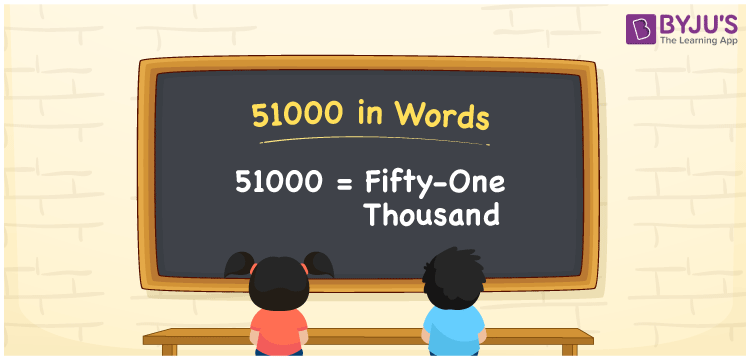# 51000 in words

51000 in words is written as Fifty One Thousand. In 51000, 5 has a place value of ten thousand and 1 has the place value of thousand. The article on Place Value gives more information. The number 51000 is used in expressions that relate to money, distance, length, population and many more. For example, “A new township has Fifty One Thousand people.” We can also use 51000 as “A Mango farm area was 5.1 hectares. It means the area was 51000 sq meters.”

 51000 in words Fifty One Thousand Fifty One Thousand in Numbers 51000

## 51000 in English Words## How to Write 51000 in Words?

We can convert 51000 to words using a place value chart. The number 51000 has 5 digits, so let’s make a chart that shows the place value up to 5 digits.

 Ten thousand Thousands Hundreds Tens Ones 5 1 0 0 0

Thus, we can write the expanded form as:

5 × Ten thousand + 1 × Thousand + 0 × Hundred + 0 × Ten + 0 × One

= 5 × 10000 + 1 × 1000 + 0 × 100 + 0 × 10 + 0 × 1

= 51000

= Fifty One Thousand

51000 is the natural number that is succeeded by 50999 and preceded by 51001.

51000 in words – Fifty One Thousand.

Is 51000 an odd number? – No.

Is 51000 an even number? – Yes.

Is 51000 a perfect square number? – No.

Is 51000 a perfect cube number? – No.

Is 51000 a prime number? – No.

Is 51000 a composite number? – Yes.

## Solved Example

1. Write the number 51000 in expanded form

Solution: 5 x 10000 + 1 x 1000 + 0 x 100 + 0 x 10 + 0 x 1

Or Just 5 x 10000 + 1 x 1000

We can write 51000 = 50000 + 1000 + 0 + 0 + 0

= 5 x 10000 + 1 x 1000 + 0 x 100 + 0 x 10 + 0 x 1

## Frequently Asked Questions on 51000 in words

Q1

### How to write 51000 in words?

51000 in words is written as Fifty One Thousand.
Q2

### State True or False. 51000 is divisible by 4?

True. 51000 is divisible by 4.
Q3

### Is 51000 divisible by 10?

Yes. 51000 is divisible by 10. It is also divisible by 2, 3, 4, 5, 6, 10, 100 and 1000.